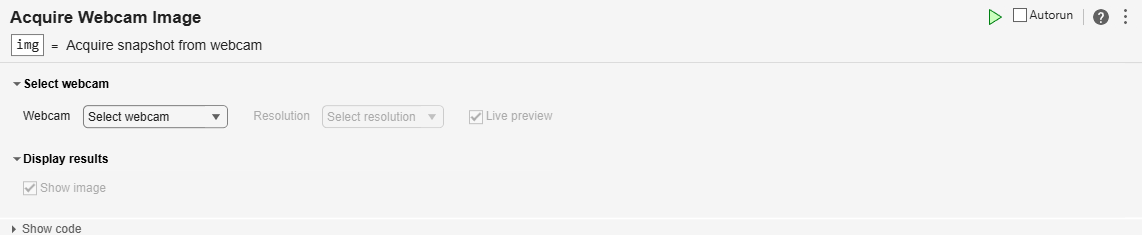# Detect and Plot Water Level Change with USB Webcam Live Editor Task

This example shows you how to set up your USB webcam to detect and plot the change in water level. Interactively capture images from a USB webcam using the Acquire Webcam Image task in the Live Editor. For more information on Live Editor tasks, see Add Interactive Tasks to a Live Script.

In this example, you will estimate the level of water in a cup using image subtraction to subtract sample images from a reference image. You will also plot the normalized water levels in real-time. Use the Acquire Webcam Image task in a loop to capture the sample images.

This example uses the reference image `image01.png`.

`refImage = imread("image01.png");`

This image is of an empty glass with a resolution of 640 x 480.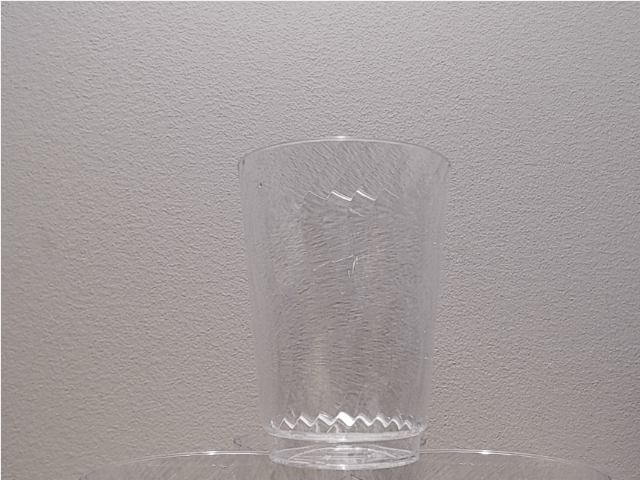Alternatively, you can capture this image using the Acquire Webcam Image task.

### Set up Output Window

Set up the figure to plot the water level changes in real time.

```fig = figure("Name",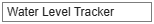"Water Level Tracker","Visible","off"); t = tiledlayout(2,2,"Parent",fig); t.Padding = 'none'; t.TileSpacing = 'none'; ax1 = nexttile(t); hold (ax1,'on'); title("Reference Image","Parent",ax1); imshow(refImage,"Parent",ax1); ax2 = nexttile(t,[2 1]); title('Water level v/s Time',"Parent",ax2); xlabel(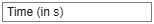'Time (in s)',"Parent",ax2); ylabel(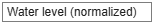'Water level (normalized)',"Parent",ax2); ax3 = nexttile(t); title("Water Level","Parent",ax3); grid on; h = animatedline(ax2,"Color",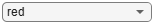"red","LineWidth",3); webcamLETFig = figure("Name","Acquire Webcam Image Output");```

### Plot Water Level Change

To determine the change in water level, use image subtraction. Subtract each sample image from the reference image. To capture the sample images`,`use the Acquire Webcam Image task.

Ensure the following in the Live Task:

1. Select a webcam from the webcams connected to your computer.

2. Clear the Show image check box to prevent displaying the image in each loop iteration.

3. Turn off the Autorun feature if you want the live task to execute only when the entire script is executed. To disable autorun, click the autorunicon.

4. Select Live preview to view the live video from the webcam while adjusting webcam properties.

Do the following:

1. Capture images using the Acquire Webcam Image task in a loop. You can vary the time for which the loop executes by entering the time in seconds in the textbox.

2. Subtract each `sampleImage` from the `refImage` to get the `diffImage. `

3. Extract the the RGB components from `diffImage` and convert the image to grayscale to reduce the noise in the image.

4. Find the mean pixel intensity value across each row of the image. The mean pixel intensity is used to find the image pixel corresponding to the top of the water level.

5. Define a window size and a threshold for the intensity by moving the slider.

6. Find the difference in the instensities between the n+10th pixel and nth pixel in the `windowSize`. 'n' is the row index and 10 is the window size used in this example. If the intensity of the pixel value is greater than the threshold intensity, store the pixel value as the water level.

7. Plot the water level against time.

The live editor task` Acquire Webcam Image` is as shown.` `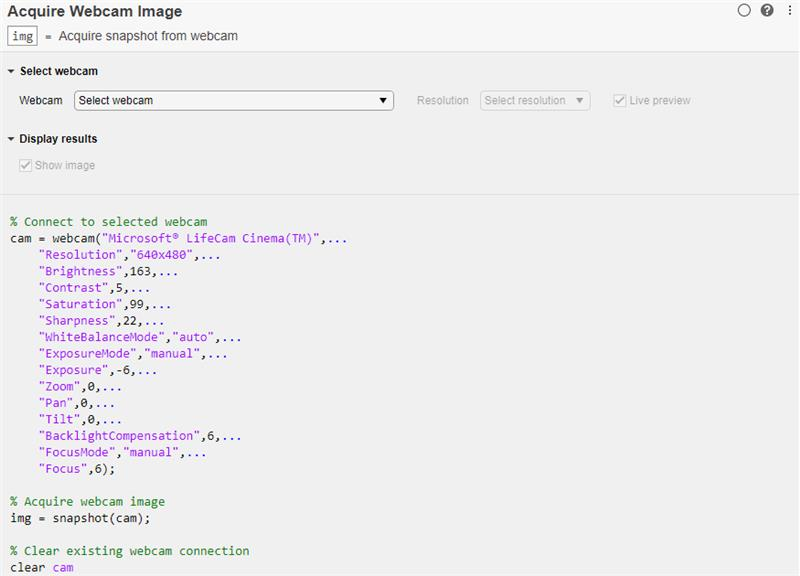You can set the properties of the webcam as shown in this image.When the glass is empty, you will see the level is at zero.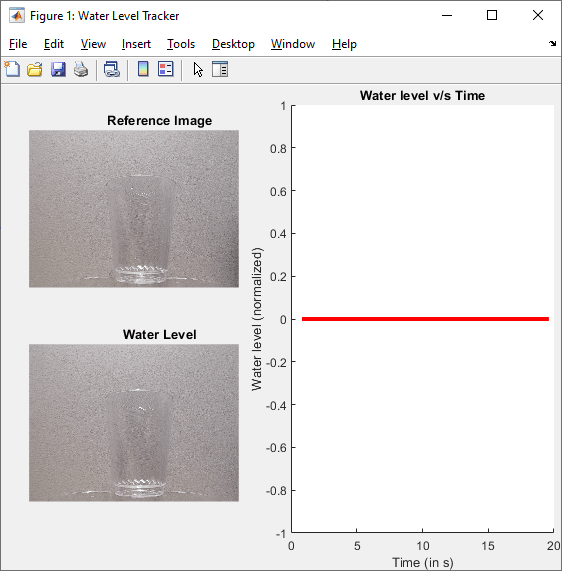```% Start time-stamp timer for plotting water level against time t = tic; while(toc(t) <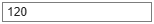120) % Read the image captured from the webcam % Make the "Acquire Webcam Image Output" figure the active figure figure(webcamLETFig);sampleImage = img; % Store the relative timestamp tStamp = toc(t); % Subtract the sample image from the reference image % 'imsubtract' from the Image Processing Toolbox can be used here diffImage = refImage - sampleImage; dims = size(diffImage); % Extract RGB components from the difference image R = diffImage(:,:,1); G = diffImage(:,:,2); B = diffImage(:,:,3); % Convert the difference image to grayscale greyDiffImage = zeros(dims(1),dims(2),'uint8'); for row = 1:dims(1) for col = 1:dims(2) greyDiffImage(row,col) = (0.2989 * R(row,col)) + (0.5870 * G(row,col)) + (0.1140 * B(row,col)); end end % Find mean pixel intensity value across each row of the image rowMean = zeros(1,dims(1)); for row = 1: dims(1) rowMean(row) = mean(greyDiffImage(row,:)); end % Find the image pixel corresponding to the top of water level % Find the difference instesities between the n+10th and nth pixel. % Store this pixel value as the water level, if this intesnity is greater than a threshold windowSize =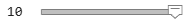10; intensityThreshold =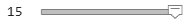15; waterLevelPixel = dims(1); for rowIndex = 1:length(rowMean)-windowSize if rowMean(rowIndex+windowSize) - rowMean(rowIndex) > intensityThreshold waterLevelPixel = rowIndex; break; end end % Display images % Make the "Water Level Tracker" figure the active figure figure(fig); % Display the reference image % Display the current water level hold(ax3, 'all'); imshow(sampleImage,"Parent",ax3); quiver(dims(2)/2,waterLevelPixel,0,dims(1)-waterLevelPixel,... "Color",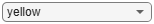"yellow","LineWidth",3,'Parent',ax3); hold off; % Plot water level vs/ time addpoints(h,tStamp,abs(1 - (waterLevelPixel/dims(1)))); drawnow; fig.Visible = "on"'; end```